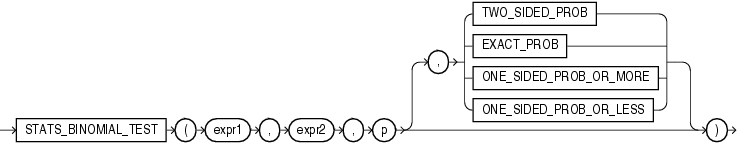# STATS_BINOMIAL_TEST

SyntaxDescription of the illustration stats_binomial_test.gif

Purpose

`STATS_BINOMIAL_TEST` is an exact probability test used for dichotomous variables, where only two possible values exist. It tests the difference between a sample proportion and a given proportion. The sample size in such tests is usually small.

This function takes four arguments: `expr1` is the sample being examined. `expr2` contains the values for which the proportion is expected to be, and `p` is a proportion to test against. The fourth argument is a return value of type `VARCHAR2`. If you omit the fourth argument, then the default is `TWO_SIDED_PROB`. The meaning of the return values is shown in Table 5-3.

Table 5-3 STATS_BINOMIAL Return Values

Return Value Meaning

`TWO_SIDED_PROB`

The probability that the given population proportion, `p`, could result in the observed proportion or a more extreme one.

`EXACT_PROB`

The probability that the given population proportion, `p`, could result in exactly the observed proportion.

`ONE_SIDED_PROB_OR_MORE`

The probability that the given population proportion, `p`, could result in the observed proportion or a larger one.

`ONE_SIDED_PROB_OR_LESS`

The probability that the given population proportion, `p`, could result in the observed proportion or a smaller one.

`EXACT_PROB` gives the probability of getting exactly proportion p. In cases where you want to test whether the proportion found in the sample is significantly different from a 50-50 split, `p` would normally be 0.50. If you want to test only whether the proportion is different, then use the return value `TWO_SIDED_PROB`. If your test is whether the proportion is more than the value of `expr2`, then use the return value `ONE_SIDED_PROB_OR_MORE`. If the test is to determine whether the proportion of `expr2` is less, then use the return value `ONE_SIDED_PROB_OR_LESS`.

STATS_BINOMIAL_TEST Example The following example determines the probability that reality exactly matches the number of men observed under the assumption that 69% of the population is composed of men:

```SELECT AVG(DECODE(cust_gender, 'M', 1, 0)) real_proportion,
STATS_BINOMIAL_TEST
(cust_gender, 'M', 0.68, 'EXACT_PROB') exact,
STATS_BINOMIAL_TEST
(cust_gender, 'M', 0.68, 'ONE_SIDED_PROB_OR_LESS') prob_or_less
FROM sh.customers;
```